Journal of Finance and Accounting
Volume 4, Issue 4, July 2016, Pages: 225-233

The Effect of Market Risk on Financial Performance of Commercial Banks in Kenya

Jane Gathigia Muriithi1, *, Willy Mwangi Muturi1, Kennedy Munyua Waweru2

1Department of Economic, Accounting and Finance, Jomo Kenyatta University of Agriculture and Technology, Nairobi, Kenya

2Department of Finance and Accounting, Co-operative University College of Kenya, Nairobi, Kenya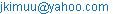(J. G.Muriithi)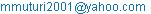(W. M. Muturi)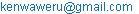(K. M. Waweru)

*Corresponding author

Jane Gathigia Muriithi, Willy Mwangi Muturi, Kennedy Munyua Waweru. The Effect of Market Risk on Financial Performance of Commercial Banks in Kenya. Journal of Finance and Accounting. Vol. 4, No. 4, 2016, pp. 225-233. doi: 10.11648/j.jfa.20160404.18

Received: June 17, 2016; Accepted: July 7, 2016; Published: July 23, 2016

Abstract: Despite the growth in the Kenyan banking sector, market risk still remains a major challenge. The objective of study was to assess the effect of market risk on financial performance of commercial banks in Kenya. The study covered the period between year 2005 and 2014. Market risk was measured by degree of financial leverage, interest rate risk and foreign exchange exposure while financial performance was measured by return on equity. The study used the balance sheets components and financial ratios for 43 registered commercial banks in Kenya. Panel data techniques of random effects, fixed effects estimation and generalized method of moments (GMM) were used to purge time–invariant unobserved firm specific effects and to mitigate potential endogeneity problems. The pairwise correlations between the variables were carried out. F- test was used to determine the significance of the regression while the coefficient of determination, within and between R2, were used to determine how much variation in dependent variable is explained by independent variables. From the results financial leverage, interest rate and foreign exchange exposure have negative and significant relationship with bank profitability. Based on the study findings, it is recommended that commercial banks especially locally owned are required to consider finding ways of mitigating the market risks by use of financial instruments such as financial derivatives and be active in derivatives markets. These may reduce their interest rate risk and foreign currency risk exposure. The commercial banks are also required to monitor the financial leverage so as to reduce the financial risk.

Keywords: Market Risk, Financial Performance, Commercial Banks, Degree of Financial Leverage, Interest Rate Risk, Foreign Exchange Exposure, Kenya

1. Introduction

The banking sector is the backbone of the Kenyan economy and it is a critical vehicle that links the Kenyan economy to the rest of the world. In the process of providing financial services, banks may be affected by various kinds of financial risks among them being market risk. Market risks can lead to significant losses very quickly in volatile market conditions and also complete institutional collapse in severe situations. Market risk refers to the risk to an institution resulting from movements in market prices, in particular, changes in interest rates, foreign exchange rates, and equity and commodity prices. Form of market risk also arises where banks accept financial instruments exposed to market price volatility as collateral for loans .

As the banking sector continues to embrace innovations, the intensity and variety of risks that the players are exposed also continue to increase in tandem. To ensure that the growth in the banking sector does not jeopardize its stability, risk management is crucial. A 1995 survey of major financial firms in Unites States of America (USA) revealed that at least 90% are using some form of financial engineering to manage market risks which are interest rates, foreign exchange or commodity price risks . Banks, insurance firms, savings and loans firms are also active in derivatives markets. There is substantial commonality in the underlying rationale for the use of derivatives and the financial engineering techniques that are employed although the types of risks confronting managers vary across industries. The Basel 1 Amendment in 1996 also covered market risks in the trading book which was an indication that market risk exist and is a threat to the banking sector and country’s economy as a whole.

Market risk which comprises of exchange rate, inflation and interest rate risks affect the financial performance of banks. Usually, market risks are outside the control of the banks, as they are determined by factors that affect the overall economy . Degree of financial leverage, foreign exchange rate exposure and interest rate risk were used as indicators of market risk. Degree of financial leverage (DFL) is best used to help a company determine financial leverage risk. It is practically a measure of the degree of financial risk, thus the higher the ratio is the more risky the business is considered to be as it relies too much on debts and any changes within the economic environment or in interest rates may have an extremely negative impact on how the business evolves.

Currency-related gains and losses can have destructive impacts on reported earnings which are fundamental to the markets opinion of that company. The foreign exchange rate exposure of a firm is a measure of the sensitivity of its cash flows to changes in exchange rates. Since cash flows are difficult to measure, most researchers have examined exposure by studying how the firm’s market value, the present value of its expected cash flows, responds to changes in exchange rates. Foreign exchange exposure is defined as the degree to which a company is affected by exchange rate changes. The magnitude of the gain or loss that results from a particular exchange rate change is transaction exposure which refers to foreign exchange loss or gain on transaction already entered into and denominated in a foreign currency. The study applied the unrealized foreign exchange gains and losses as proxy of foreign exchange exposure as used by reference .

The study captured the effect of interest rate as a measure of market risk since a change in interest rate could lead to a mismatch between interest paid on deposit and the interest received on loans. The interest rate risk is proxied by Net Interest Margin (log of NIM), and it is adjusted for change in interest rate as used by reference .

2. Literature Review

2.1. Extreme Value Theory

Market risk is a dominant source of income fluctuations in financial institutions all over the world. The financial institutions with significant amounts of trading activity proved to be very vulnerable to extreme market movements and, in time, the measurement of market risk became a primary concern for regulators and also for internal risk control. This calls for indicators showing the risk exposure of firms and the effect of risk reducing measures. Value-at-Risk (VaR) has been established as a standard tool among financial institutions to depict the downside risk of a market portfolio. It measures the maximum loss of the portfolio value that will occur over some period at some specific confidence level due to risky market factors . Banks and bank holding companies with an important trading portfolio are subject to market risk requirements. They have been required to hold capital against their defined market risk exposures, and, the necessary capital is a function of banks' own risk estimates.

As a result, several alternative methods have been proposed for estimating VaR, one of which being the Extreme Value Theory (EVT). EVT methods make VaR estimations based only on the data in the tails as opposed to fitting the entire distribution and can make separate estimations for left and right tails . Proper estimation of VaR is necessary in that it needs to accurately capture the level of risk exposure that the firm is exposed to, but if it overestimates the risk level, then the firm will set unnecessarily set aside excess capital to cover the risk, when that capital could have been better invested elsewhere . Hence the banks need to determine the minimum and the maximum capital that should be set aside cover the market risks. To achieve this goal the banks need to manage the market risks using the financial ratios such as degree of financial leverage. The foregoing instigates the question of effect of market risks on the financial performance of the commercial banks.

2.2. Market Risks and Financial Performance

According to the classification of banking risks introduced by foreign economists reference , market risk can be generally said to consist of three lesser risks: stock price risk, interest rate risk and foreign exchange risk. According to reference  form of market risk also arises where banks accept financial instruments exposed to market price volatility as collateral for loans. Reference  explained that price fluctuations or volatility increases and decreases in the day-to-day market. This type of risk mainly applies to both stocks and options and tends to perform well in a bull (increasing) market and poorly in a bear (decreasing) market. Generally, the more volatility within the market, the more probability there is that the investment will increase or decrease. Market risks may be divided into interest rate risks and exchange rate risks including gold, share price risks and commodity price risks which refer to respectively the risks created by any adverse change in interest rates exchange rates share prices and commodity prices.

Reference  carried a survey to identify the market risk management techniques used by commercial banks in Kenya and their suitability in mitigating financial loss. The research design adopted in the study was a census survey. The population used consisted of the 43 commercial banks licensed to operate in Kenya as listed by the Central Bank of Kenya. Primary data collection through the use of a questionnaire was used to gather information from the target population outlining issues relevant to the study. The results of the study showed that the main techniques used were Scenario analysis and Stress Testing to a very large extent. The major finding was that limits ensured management of risk exposure within the bank's risk appetite. Other reasons were limits ensured banks took acceptable limits as approved by the shareholders and there was prudent management of market risk. Other minor reasons were to ensure prudent management of the bank's assets and liabilities and for monitoring purposes . The study concluded that it is imperative that banks in Kenya pick out best practices from each in order to put market risk exposure under control to mitigate the effects of losses due to this risk. The current study is different from past studies as secondary data obtained from banks annual financial reports was used in analysis.

Reference  examined the impact of systematic risk management on profitability of selected financial institutions in Sri Lanka from year 2007 to 2011. In their study, systematic risk management measured in Degree of Financial leverage (DFL) and Degree of Operating leverage (DOL) as independent variable and Profitability (i.e., Net Profit, Return on Capital Employed (ROCE) and Return on Equity (ROE) as the dependent variable. The study used secondary data. Operational hypotheses were formulated and results revealed that systematic risk management has a positive association (r= 0.755, p<0.05) with profitability. The study also indicated that systematic risk management is enhanced by DFL and DOL in the selected financial institutions where the beneficial impacts are observed on profitability.

Reference  also studied on the effect of foreign exchange exposure on a firm’s financial performance of a case of 32 selected listed companies in the Nairobi Stock Exchange. The data analysed was for the period covering years 2001 to 2010. From the study it was found that listed firms use the income statement and the owner‘s equity account to record foreign exchange differences. The study concluded that unrealized foreign exchange gains/losses had an effect on the Net Income of listed companies as it was posted to either income statement or owners‘equity. For comprehensive analysis of market risk, the interest rate risk needed to be incorporated in the analysis. In addition, the current study also considered the degree of financial leverage which is applied to manage the systematic risks.

Reference  showed that bank’s exposure to interest rate risk or income gap determines the structure of the balance sheet. They analyzed interest rate sensitivity gaps obtained from financial reports for 10 commercial banks listed in the Nairobi securities exchange for the period 2008-2012. In particular, they found that in Kenya, commercial banks typically retain a large exposure to interest rates that can be predicted through the income gap. They also established the sensitivity of income gaps to market interest rates as determined by the CBK through treasury instruments. Quantitatively, a 200 basis point change in CBK rates would lead to a change of net income equivalent to 0.4% of total assets of the bank. They recommended further research on wider sample of banks over a longer time series period to establish a comprehensive effect of interest risk exposure on Kenyan financial performance.

3. Methodology

3.1. Research Design

The research study employed Time Series Cross Sectional (TSCS) research design that was used to show the effect of the financial risks on the financial performance of commercial banks in Kenya. (TSCS) research design is a quasi-experimental research design that reference  explained that TSCS designs have long been considered as one of the best designs for the study of causation, next to a purely random experiment. Reference  states that TSCS designs are research designs "par excellence." In addition to their potential for detecting causal relationships, TSCS designs offer a number of distinct advantages. The study used financial ratio analysis and unbalanced panel data regression analysis to measure, describe and analyse the effect of the financial risks on the financial performance of commercial banks in Kenya during the period 2005-2014. Panel data estimation techniques of fixed effects estimation and generalized method of moments (GMM) was adopted because it takes care of heterogeneity associated with individual banks by allowing for individual specific variables.

The researcher employed a census study. Hence the secondary data for all the 43 licensed commercial banks bank in Kenya was collected and analyzed. The long run specification was estimated using the fixed effects or random effects models while the short run model was estimated using system Generalized Methods of Moments (GMM) estimator as put forward by reference . The estimation of the short run model was preceded by the estimation of the naïve OLS and fixed effects models inorder to establish the required bound for coefficient of lagged bank performance as put forth by reference .

Multicollinearity is a typical phenomenon in time-series data that refers to the tendency of the explanatory variables to co-vary and hence making it difficult to determine the statistical significance of each independent variable. To establish the satisfaction of pre-estimation assumptions of tolerable multicollinearity and normality of the one way error component models, correlation analysis and extended Bera-Jarque normality test by reference  were to be used.

To establish the reliability of the estimates a number of post estimation diagnostics were to be interpreted. The fixed and random effect model involved interpretation of the F statistic, interclass correlation (rho), within and between R-square, chow test statistics, LM test statistic and Hausman test. The short run GMM specification involved the interpretation of Hansen J statistic and the Arrelano and Bond autocorrelation tests

3.2. Model Specification

The objective of this study was to establish whether market risk affects the financial performance of the commercial banks in Kenya. The study assumed that the independent variables and the dependent variable have a general multiplicative Cobb Douglas functional relationship shown in equation 3.1.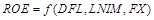(1)

Upon linearization and parametization the long run model for functional form 3.1 was specified as: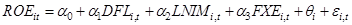(2)

And the short run model as: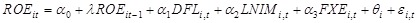(3)

In which ROEi,t represents the performance of Bank i at time t,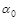stands for the model constant or intercept,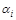stands for the coefficients of the independent variables.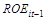is lagged bank performance, DFLi,t is the Degree of Financial Leverage of bank i at time t, interest rate risk is proxied by log of Net Interest Margin (NIM), LNIMi,t for bank i at time t and FXEi,t foreign exchange exposure risk is proxied by log of foreign exchange gains/loss.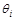is the bank specific effect that is assumed to be normally distributed with a constant variance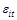is the idiosyncratic error term which is assumed to have a normal distribution.

The linearization process involved logging the variables. Therefore, all the variables were to enter models 2 and 3 in log form. This inherently made theand the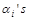elasticities.

3.3. Summary Statistics of Data

Table 1. Summary Statistics for the Data Set.

 Variables N Mean Standard Deviation Min Max DFL 416 3.042 4.700 2.340 54.854 NIM 413 0.520 6.678 0.224 127.02 FX 405 6.446 0.768 5.314 11.400

From the table 1 the average DFL of Kenyan banks was 3.042 with standard deviation of 4.700. The maximum and minimum values were 2.340 and 54.854 respectively. There was high degree of financial level (DFL) during the study period which is an indication that employing heavy financial leverage which is allowing the commercial banks to greatly expand earnings per share as a result of a change in earnings before interest and taxes.

Further table 1 shows that the mean net interest margin was 52.0 percent with a corresponding standard deviation of 6.678. Therefore, this implies the difference between the interest income and interest expenses are high for commercial banks and most of them are expected to be profitable. The mean of net foreign currency exposure was 6.446 percent with a corresponding standard deviation of 0.768. Therefore, there is risk of unexpected changes in foreign exchange currency rates on Kenyan commercial banks.

3.4. Correlation Between Market Risk’s Dimensions and Return on Equity

Market risk had three consisted of degree of financial leverage (DFL), net interest margin (NIM) and foreign currency exchange exposure (FXE). The correlation between these dimensions themselves and return on equity is shown in table 2 below.

Table 2. Correlation between Market Risk Components and Return on Equity.

 ROE DFL NIM NFCE ROE 1 DFL -0.764 1 (0.000) NIM -0.597 0.253 1 (0.000) (0.000) FXE -0.421 0.213 0.671 1 (0.000) (0.000) (0.000)

Key: P-values in parenthesis

Table 2 shows that all the dimensions of market risk are significantly negatively correlated to return on equity. This implies that market risk is negatively associated with financial performance of commercial banks in Kenya. This is expected since changes in lending and foreign exchange rates move in different directions with bank performance. The fact that dimensions of market risk are negatively associated with return on equity means that the coefficients of the dimensions in the regressions were expected to be negative.

From table 2 the correlation coefficient between ROE and net interest margin which is a proxy of interest rate risk was found to be significant and negative. The correlation coefficient is negative 0.597 with a corresponding p-value of 0.000. Therefore, it is significantly different from zero at one per cent level of significance. Increase in market interest rates causes the banks to increase their lending rates where the borrowers may default and hence low profits. Low interest rates may influence bank risk by affecting the valuation, cashflows and also the income streams of the banks. The banks also shift the cost on loan default to other customers in the form of higher interest rate on loans. Higher interest margin charged on loan by commercial banks due to weak credit risk management practices prevent customers and micro-enterprises from accessing loans. Such a situation prevents business expansion and affects the return expected by the shareholders.

The correlation coefficient between foreign exchange exposures with net interest margin is significant and positive. The correlation coefficient is 0.671 with a corresponding p-value of 0.000. Therefore, it is significantly different from zero at one per cent level of significance. This may be explained by the fact that changes in interest rates are used to stabilize the exchanges rate by the central bank in Kenya. Thus depreciation in the shilling is associated with a higher interest margin. The connection between foreign exchange exposure with net interest margin is purely interventional not causational.

3.5. Skewness, Kurtosis and Normality Test of One Way Error Component for Panel Models

The extension of the Bera-Jarque normality test by reference  made the normality test a standard test that can be performed prior to the estimation of the model or even after the estimation of the model. The normality test of each of the components in the error term is shown in table 3 for each model.

Table 3. Skewness Kurtosis and Normality of One way Error component for Panel Models.

 Skewness Kurtosis Normality Model Error Component Z Statistic P-Value Z Statistic P –Value Chi Statistic P-Value 3.1 e -0.5 0.614 2.48 0.0873 6.4 0.0576 u 0.09 0.93 -0.84 0.402 0.71 0.7008

Table 3 shows the distribution of the one way error component in the linear panel model. The individual specific heterogeneity component is u while the rest of the error term is e. u varies with banks only while e varies across banks and time. To use the variance of the combined error term to test the significance of the coefficients in the estimates of the model requires that each component is normally distributed. Therefore, the skewness and kurtosis of the components should be symmetrical to that of the normal distribution.

Table 3 shows that the z statistic for the skewness of all the components in model 1 have z statistics with corresponding p-values that are greater than 0.01. Thus the Z statistics are less than the tabulated at five per cent level of significance. Therefore, the null hypothesis of symmetrical skewness with normal distribution is not rejected for any component in all the models. Thus the components are neither negatively nor positively skewed compared to the normal distribution.

Table 3 further shows that the z statistics for kurtosis of all the components of the error terms in model 1 have p-values greater than 0.1. Therefore the z statistics are less than the tabulated statistics at five per cent level of significance. Thus the null hypothesis that each components kurtosis is symmetric to that of the normal distribution is not rejected at five per cent level of significance. Therefore the components of the error term are neither more nor less peaked than the normal distribution.

The overall normality test of each component of the error term in model 1 has chi statistics with corresponding p-value that are greater than 0.1. Therefore, the chi statistics are less than the critical values at five per cent level of significance. Thus the null hypothesis that each component is normally distributed is not rejected at five per cent level of significance for all the models. Therefore the error components are normally distributed for model 1.

3.6. Empirical Findings

The study presents the findings as follows; (1) each long run model is presented separately and its post-estimation diagnostics discussed to establish the reliability of the findings (2) the study discriminates between the long run models using Hausman test (3) the study presents the naïve OLS and fixed effects estimates of the short run specification to establish the range where the coefficient of lagged return on equity should lie in the GMM specification (4) the study estimates and presents the GMM specification while presenting the instruments used and discussing the post-estimation diagnostics of the GMM model. Finally (5) the study presents a comparative summary of all the models and tests the hypotheses both in the short and in the long run.

To test the hypothesis the long run and the short run version of model 1 were estimated. The long run specification consisted of the fixed and random effects model. The fixed effects estimates are shown in table 4.

Table 4. Model 1 Fixed Effects Estimates.

 Dependent variable ROE Explanatory Variable Coefficient DFL -0.626*** NIM -0.174*** FXE -0.139*** Constant -0.886*** Post Estimation Diagnostics R square Within 0.5951 Between 0.7860 Overall 0.7155 Rho 0.6084 F test (3, 322) 157.74*** chow test F (41, 322) 8.19*** KEY p-value <0.01 *** P-value <0.05 ** P –value<0.1 *

Table 4 shows that the F statistic is 157.74 and is greater than the critical value at one per cent level of significance. Therefore, the variables (market risk components) are jointly significant in explaining the variations in return on equity.

The interclass correlation (rho) is 60.84 per cent implying that 60.84 per cent of the variations in return on equity are due to differences across the banks. The within and between R-square is 59.51 per cent and 78.60 per cent respectively. Thus, 59.51 per cent of variations in the return on equity are due to differences within individual banks and 78.60 per cent of the variations are due to differences between the banks.

The chow test statistic is 8.19 and is greater than the critical value at one per cent level of significance. Therefore, the null hypothesis that the fixed effects are equal to zero is rejected at one per cent level of significance. Thus the option of specifying the long run version of model 2 as a pooled OLS model over the fixed effects specification is rejected at one per cent level of significance.

The alternative long run specification of model 1 were the random effects model. The estimates for this specification are shown in table 5.

Table 5. Model 1 Random Effects Estimates.

 Dependent variable ROE Explanatory Variable Coefficient DFL -0.643*** NIM -0.257*** FXE -0.120*** Constant -3.182*** Post Estimation Diagnostics R square Within 0.5931 Between 0.7976 Overall 0.7260 Rho 0.5010 Wald test (3, 365) 606.91*** Lm test Chibar 2 172.63*** KEY p-value <0.01 *** P-value <0.05 ** P –value<0.1 *

Table 5 shows that the Wald statistic is 606.91 and is greater than the critical value at one per cent level of significance. Therefore, the variables (market risk components) are jointly significant in explaining the variations in return on equity in the random effects specification.

The interclass correlation (rho) is 50.10 per cent implying that 50.10 per cent of the variations in return in equity are due to differences across the banks as per the random effects model. The within and between R-square is 59.31 per cent and 79.76 per cent respectively. Thus, 59.31 per cent of variations in the return on equity are due to differences within individual banks and 79.76 per cent of the variations are due to differences between the banks.

The LM test statistic is 172.63 and is greater than the critical value at one per cent level of significance. Therefore, the null hypothesis that the cross sections are homogeneous is rejected at one per cent level of significance. Thus the random effects specification is preferred over POLS.

A comparison of the fixed and random effects specification reveals that the two long run models lead to similar conclusions. For instance, POLS specification is rejected when compared to the fixed and random effects specification. In addition, the overall explanatory powers of the fixed and random effects specifications are not significantly different; the fixed effect specification explains an overall explanation 71.55 per cent while the random effects model has an overall explanation of 72.60 per cent. However, Hausman test is conducted to determine which model should be interpreted in the long run. The test statistic for this test is shown in table 7.

Table 6. Model 1 Hausman Test.

 Test statistic Chi (3) P-value 15.95 0.0012

Table 6 shows that the test statistics have a chi statistic of 15.95 with three degrees of freedom and a corresponding p value of 0.001. Therefore, the null hypothesis that the regressors and individual heterogeneity are strictly exogenous is rejected at one per cent level of significance. Thus the FE specification is preferred over RE specification. Therefore, for the long run specification the fixed effects model should be interpreted.

To establish the bound where the coefficient of lagged profits would lie in the short run specification of model 1 the naïve OLS was estimated to establish the upper bound of the coeffient. The OLS estimates are shown in table 7.

Table 7. Short run OLS Estimates for Model 1.

 Dependent variable ROE Explanatory Variable Coefficient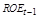0.277*** DFL -0.572*** NIM -0.368*** FXE -0.581*** Constant -2.778*** Post Estimation Diagnostics R squared 0.7954 F statistic (4, 320) 310.94*** KEY p-value <0.01 *** P-value <0.05 ** P –value<0.1 *

Table 8 shows that the coefficient of lagged return on equity is 0.277. Therefore, the upper bound for the coefficient of lagged return on equity in the GMM specification of the short run model should be 0.277. To get the lower bound the fixed effect estimates of the short run specification of model 1 are used. The estimates of the short run specification are shown in table 8.

Table 8. Short run Fixed Effects Estimates for Model 1.

 Dependent variable ROE Explanatory Variable Coefficient0.078** DFL -0.665** NIM -0.198*** FXE -0.429*** Constant 1.030 Post Estimation Diagnostics R squared 0.6186 F statistic (4, 314) 113.14*** KEY p-value <0.01 *** P-value <0.05 ** P –value<0.1 *

Table 8 shows the fixed effects estimates of the short run specification of model 1. The coefficient of lagged return on equity is 0.078. Thus the lower bound of lagged return on equity in the GMM specification should be 0.078. Specifically if the estimate is, it should lie in the interval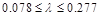. The one step system GMM consistent estimates of the short run spefication are shown in table 9.

Table 9. Model 1 One Step System GMM Estimates.

 Dependent variable ROE Explanatory Variable Coefficient0.138*** DFL -0.646*** NIM -0.447*** FXE -0.658*** Constant 3.290*** Post Estimation Diagnostics Hansen J test 40.21 AR (1) -1.54** AR (2) -2.27 KEY p-value <0.01 *** P-value <0.05 ** P –value<0.1 *

Table 9 shows the one step system GMM estimates for the short run specification of model 1. The table shows that the coefficient of the lagged return on equity is 0.138. The coefficient, therefore, lies in the acceptable range ofetablished by the naïve OLS estimates and fixed effects estimates of the short run model 1. This points to consistency of estimates.

Table 9 further shows that the Hansen J statistic is 40.21 with a corresponding p-value greater than 0.1. Therefore, the null hypothesis of the validity of the overidentifying restrictions for the instruments is not rejected at one per cent level of significance. Therefore, the instruments employed by the model are appropriate and lead to precise consistent estimates.

In addition table 9 shows that the test of autocorrelation in the error terms. The AR (1), first order autocorrelation, test statistic is -1.54 and is greater than the critical value at five per cent level of significance. Therefore, the null hypothesis that disturbance term (error terrm) has no first order serial correlation is rejected at one per cent level of significance. This is expected because of the dynamic specification of model 2 and therefore, points to correct specification. The test statistic for second order serial correlation in the error term is -2.27 with a coreresponding p-value that is greater than 0.1. Therefore, at one per cent level of significance the null hypothesis that there is no second order serial correlation in the disturbance term is not rejected at one per cent level of significance permiting the use of insturments from the second lag and differences. This further supports the argument of correct short run specification of model 1 using the one step GMM estimates.

To Summarize the findings necessary to test the second hypothesis in the short run and in the long run. The findings in table 4 through table 9 are summarized in table 10.

Table 10. Effect of Market Risk on Financial Performance of Commercial Banks in Kenya.

 Long Run Estimates Short Run Estimates Variables Random Effects Fixed Effects Naïve OLS Fixed Effects GMM0.278*** 0.0777** 0.138** (0.036) (0.0360) (0.057) DFL -0.643*** -0.626*** -0.572*** -0.665*** -0.646*** (0.030) (0.031) (0.036) (0.035) (0.062) NIM -0.257*** -0.174*** -0.368*** -0.198*** -0.447*** (0.050) (0.0575) (0.0471) (0.064) (0.085) FXE -0.120*** -0.139*** -0.581*** -0.429 -0.658** (0.042) (0.045) (0.198) (0.310) (0.322) Constant 1.361*** 0.886** 2.778*** 1.030 3.290*** (0.312) (0.359) (0.599) (0.844) (1.020) Observations 367 367 325 325 325 R-squared 0.595 0.795 0.622 Hausman Chi (3) 15.95** Wald statistic 606.91*** F statistic 157.74*** 310.94*** 113.14*** 110.73***

Key: Standard errors in parentheses

*** p<0.01, ** p<0.05, * p<0.1

Table 10 shows the long run and short run estimates on the effect of market risk on financial performance of commercial banks in Kenya. The estimates are comparable in terms signage but differ on the magnitude of coefficients. It shows that in the long run the coefficient of degree of financial leverage is -0.626 with a p value less than 0.01. Thus, the coefficient is significantly different from zero at one per cent level of significance. Therefore, the null hypothesis that degree of financial leverage has a negative significant effect on financial performance of commercial banks in Kenya is not rejected at one per cent level of significance. The magnitude of the coefficient is 0.626. Since the dependent variable, ROE, as well as degree of financial leverage enter model 1 in log form, a one per cent increase in degree of financial leverage decreases return on equity by 62.6 percentage points in the long run holding other factors constant.

In the short run the coefficient of degree of financial leverage is -0.646 with a p-value less than 0.01. Therefore, the coefficient is significant at one per cent and negative. Thus in the short run the null hypothesis that degree of financial leverage has a significant negative effect on financial performance of commercial banks in Kenya is not rejected at one per cent level of significance. The magnitude of the coefficients is 0.646. The fact that return on equity and degree of financial leverage enter the equation in log form implies that the coefficient is an elasticity. Thus in the short run a one per cent increase in degree of financial leverage causes a 64.6 percentage points decrease in the financial performance of commercial banks in Kenya holding other factors constant.

The degree of financial leverage measures the proportion of the earnings before interest and taxes against the earnings before taxes which shows the debt amount that a business is obligated to pay back. Therefore as interest increases, financial leverage will increase. Interest, in turn, being the cost of borrowed funds, will increase with increase in the proportion of debt used for financing assets. The higher the degree of financial leverage of a firm, the greater is the sensitivity of its profits before tax to changes in profits before interest and tax. This result is different from finding of reference  that there exist a moderate positive association between degree of financial leverage and return on equity. The divergence may be as result of the scope and period of the study. Also Sri Lanka and Kenya operates in two different economies.

Table 10 additionally shows that in the Long run the coefficient of net interest margin is -0.174 with a p value less than 0.01. Thus, the coefficient is significantly different from zero at one per cent level of significance. Therefore, the null hypothesis that net interest margin has a significant negative effect on financial performance of commercial banks in Kenya is not rejected at one per cent level of significance. The magnitude of the coefficient is 0.174. Since the dependent variable, return on equity, as well as net interest margin enter equation 2 in log form, one per cent increase in net interest income decreases return on equity by 17.4 percentage points in the long run holding other factors constant.

In the short run the coefficient of log of net interest margin is -0.447 with a p-value greater than 0.01. Therefore, the coefficient is significant at one per cent. Therefore, the null hypothesis that net interest margin has a significant negative effect on financial performance of commercial banks in Kenya in the short run is not rejected at one per cent level of significance. The magnitude of the coefficient is 0.447. Since the dependent variable, return on equity, as well as net interest margin enter equation 3 in log form, one per cent increase in net interest income decreases return on equity by 44.7 percentage points in the long run holding other factors constant. Hence the results indicate that there is a negative association between the interest rate risk and return on equity. This association is expected because fluctuations of interest rate lend to fluctuations in net interest margins which may cause low bank profitability. The result is in line with results by  that interest rate risk affects the bank profitability negatively. This result may be due to that where floating rate assets and liabilities that reprice at similar times and have base rates of similar maturity still may involve interest rate risk. The bank is expected to be subjected to basis risk reflecting the possibility if the instruments have different base rates. This is because the two base rates will diverge unexpectedly owing to differing credit risk or liquidity characteristics that affect the bank profitability.

Table 10 also shows that in the long run foreign exchange risk has a coefficient of -0.139 with p-values less than 0.01. Therefore, the coefficient is significant at one per cent. Therefore, the null hypothesis that foreign exchange risk has a significant negative effect on financial performance of commercial banks in Kenya is not rejected at one per cent level of significance. The magnitude of the coefficient is 0.139. Since the dependent variable, return on equity, as well as foreign exchange risk enter equation 3 in log form, one per cent increase in foreign exchange risk decreases return on equity by 13.9 percentage points in the short run holding other factors constant.

In the short run the coefficient of foreign exchange risk is -0.658 with a p-value greater than 0.01. Therefore, the coefficient is significant at one per cent. Therefore, the null hypothesis that foreign exchange risk has a significant negative effect on financial performance of commercial banks in Kenya in the short run is not rejected at one per cent level of significance. The magnitude of the coefficient is 0.658. Since the dependent variable, return on equity, as well as foreign exchange exposure enter equation 3 in log form, one per cent increase foreign exchange risk decreases return on equity by 65.8 percentage points in the short run holding other factors constant.

To jointly test whether the components of market risk influenced the financial performance of commercial banks in Kenya F test was used. The test has a null hypothesis that all the coefficients of the components of market risk are jointly equal to zero. Table 10 shows that in the long run the F statistic is 157.74 and is greater than the critical value at one per cent level of significance. Therefore, in the long run null hypothesis one that market risk has a significant effect on the financial performance of commercial banks in Kenya is not rejected at one per cent level of significance. In the short run the F statistic is 110.73 and is greater than the critical value at one per cent level of significance. Thus in the short run null hypothesis two that market risk has a significant effect on the financial performance of commercial banks in Kenya is not rejected at one per cent level of significance. Thus market risk influences financial performance of commercial banks in Kenya both in the short run and in the long run. The results concur with the findings of studies by  and  that foreign exchange exposure and interest rate risk have effect on net income.

4. Conclusion and Recommendations

The objective sought to determine the effect of market risk on profitability of commercial banks. All other factors held constant only 59.5% of the variation in profitability can be explained by change in market risk. The findings revealed that market risk has a significant negative effect on the financial performance of commercial banks in Kenya both in the short run and in the long run. This implies that bank increased exposure to market risk reduces banks’ profits. From the results financial leverage, interest rate and foreign exchange exposure have and significant relationship with bank profitability. Based on the study findings, it is recommended that Kenyan commercial banks especially locally owned are required to consider finding ways of mitigating the market risks such use of financial derivatives and asset securitization which will reduce their interest rate and foreign currency risk exposure. The commercial banks also are required to monitor the financial leverage so as to reduce the financial risk.

References

1. Aruwa, S. A. S., and Musa, A. O. 2014. Risk components and the financial performance of deposit money banks in Nigeria. International Journal of Social Sciences and Entrepreneurship, 1 (11), 514-522.
2. Bodnar, G., & Marston, R. (1996). 1995 Survey of derivatives usage by US non-financial firms. George Weiss Center for International Financial Research, Wharton School, University of Pennsylvania.
3. Cornelia, E. T. (2012). The problems and prospects of management of small-scale business in Nigeria. (Doctoral dissertation, Department of management, Faculty of Business adiministration, University of Nigeria Enugu campus).
4. Diebold, F. X., Schuermann, T., and Stroughhair, J. D (2000). Pitfalls and Opportunities in theUse of Extreme Value Theory in Risk Management. Journal of Risk Finance 1 (2), 30-35
5. Gachua, N. F. 2011. The effect of foreign exchange exposure on a Firm’s financial performance: a case of listed companies in Kenya (Doctoral dissertation, KCA University).
6. Galvao, A. F., Montes-Rojas, G., Sosa-Escudero, W., and Wang, L. 2013. Tests for skewness and kurtosis in the one-way error component model. Journal of Multivariate Analysis, 122, 35-52.
7. Hull, J. (2012). Risk Management and Financial Institutions, + Web Site (Vol. 733). John Wiley & Sons.
8. Jorion, P. (1997). Value at Risk: The New Benchmark in controlling market risk. Irwin, Chicago, 1, 997.
9. Koch, T., and MacDonald, S. 2014. Bank management. Nelson Education.
10. Lempert, R. (1966). Strategies of research design in the legal impact study: The control of plausible rival hypotheses. Law and Society Review, 1, 111-132.
11. Ngalawa, J., and Ngare, P. 2013. Interest rate risk management for commercial banks in Kenya. Journal of Economics and Finance (IOSR-JEF) e-ISSN: 2321-5933, Retrieved from; www.iosrjournals.org
12. Nimalathasan, B., and Pratheepkanth, P. 2012. Systematic Risk Management and Profitability: A Case Study of Selected Financial Institutions in Sri Lanka. Global Journal of Management and Business Research, 12 (17).
13. Roodman, D. (2006). How to do xtabond 2: An introduction to difference and system GMM in Stata. Center for Global Development working paper, (103).
14. Stimson, J. A. (1985). Regression in space and time: A statistical essay. American Journal of Political Science, 914-947.
15. Verbeek, M. (2004). A guide to modern econometrics. (2nd ed). Southern Gate, Chichester, West Sussex, England Hoboken, NJ: John Wiley & Sons.
16. Wachiaya, J. 2011. A survey of market risk management techniques by commercial banks in Kenya and their suitability in mitigating financial loss (Doctoral dissertation, University of Nairobi, Kenya).
17. Worzala, E. 1995. Currency risk and international property investments. Journal of Property valuation and Investment, 13 (5), 23-38.

 Contents 1. 2. 2.1. 2.2. 3. 3.1. 3.2. 3.3. 3.4. 3.5. 3.6. 4.
Article ToolsAbstractPDF(267K)